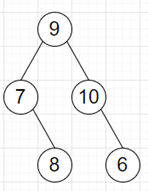# Program to find number of only child in a binary tree in python

Suppose we have a binary tree; we have to find the number of nodes that are an only child. As we know a node x is called an only child node when its parent has exactly one child that is x.

So, if the input is likethen the output will be 2 as 8 and 6 are the only children.

To solve this, we will follow these steps:

• if root is null, then
• return 0
• d := a double ended queue
• insert root at the end of d
• count := 0
• while d is not empty, do
• current := left element of d and delete left element
• if left of current is not null, then
• insert left of current into d
• if right of current is null, then
• count := count + 1
• if right of current is not null, then
• insert right of current into d
• if left of current is null, then
• count := count + 1
• return count

Let us see the following implementation to get better understanding:

## Example Code

Live Demo

from collections import deque

class TreeNode:
def __init__(self, data, left = None, right = None):
self.data = data
self.left = left
self.right = right

class Solution:
def solve(self, root):
if not root:
return 0
d = deque()
d.append(root)
count = 0
while d:
current = d.popleft()
if current.left:
d.append(current.left)
if not current.right:
count += 1
if current.right:
d.append(current.right)
if not current.left:
count += 1
return count

ob = Solution()
root = TreeNode(9)
root.left = TreeNode(7)
root.right = TreeNode(10)
root.left.right = TreeNode(8)
root.right.right = TreeNode(6)
print(ob.solve(root))

## Input

root = TreeNode(9)
root.left = TreeNode(7)root.right = TreeNode(10)root.left.right = TreeNode(8)root.right.right = TreeNode(6)

## Output

2